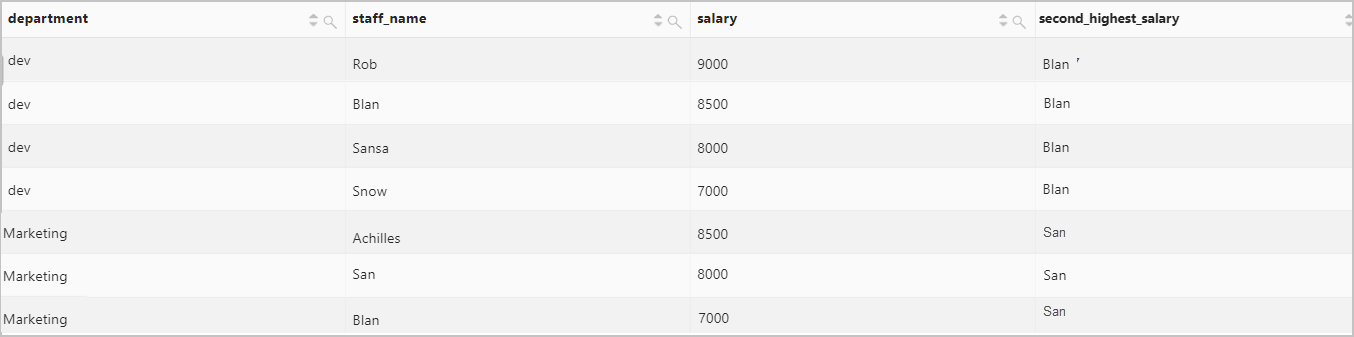This topic describes the syntax of window functions and provides examples on how to use window functions.

## Summary

Aggregate functions calculate the single result for a group of rows, and window functions calculate the result for each row in a group. A window function has three elements: partition, order, and frame. For more information, see Window Function Concepts and Syntax.

``````function over (
[partition by partition_expression]
[order by order_expression]
[frame]
)``````
• Partition: The partition element is defined by the PARTITION BY clause. The PARTITION BY clause separates rows into partitions. If you do not specify the PARTITION BY clause, all rows are treated as a single partition.
• Order: The order element is defined by the ORDER BY clause. The ORDER BY clause sorts rows in all partitions.
Note If you use the ORDER BY clause to sort rows on fields that have the same value, the order of these rows is non-deterministic. You can include additional fields in the ORDER BY clause to obtain the expected order of these rows. Example: `order by request_time, request_method`.
• Frame: The frame element is defined by the FRAME clause. The FRAME clause specifies a subset of each partition. A frame further refines the rows in each partition. You cannot specify the FRAME clause for ranking functions. Syntax of the FRAME clause: `{ rows | range} { frame_start | frame_between }`. Example: `range between unbounded preceding and unbounded following`. For more information, see Window Function Frame Specification.

## Window functions

CategoryFunctionSyntaxDescription
Aggregate functionsAggregate functionsNoneYou can use all aggregate functions as window functions. For more information about aggregate functions, see Aggregate function.
Ranking functionscume_dist functioncume_dist()Calculate the cumulative distribution of each value in a partition. The result is obtained by using division. The numerator is the number of rows whose field values are smaller or equal to the field value of the specified row. The specified row is also counted. The denominator is the total number of rows in the partition. The calculation is based on the order of the rows in the partition. Value range: (0,1].
dense_rank functiondense_rank()Calculates the rank of each value in a partition. Rows that have the same field value are assigned the same rank. The ranks are consecutive. For example, if two rows have the same rank of 1, the rank of the next row is 2.
ntile functionntile(n)Divide the rows in each partition into the number of groups specified by the N parameter.
percent_rank functionpercent_rank()Calculates the percentage ranking of each row in a partition.
rank functionrank()Calculate the rank of each row in a partition. Rows that have the same field value are assigned the same rank. The ranks are not consecutive. For example, if two rows have the same rank of 1, the rank of the next row is 3.
row_number functionrow_number()Calculate the rank of each row in a partition. The ranks are unique, and start from 1. Rows that have the same value are assigned consecutive ranks. For example, three rows with the same field value are assigned the ranks 1, 2, and 3.
Offset functionsfirst_value functionfirst_value(x)Returns the value of the specified field in the first row of each partition.
last_value functionlast_value(x)Returns the value of the specified field in the last row of each partition.
lag functionlag(x,offset,defaut_value)Returns the value of the specified field in the row that is at the specified offset before the current row of each partition. The offset is specified by the offset parameter. If no row exists at the specified offset before the current row, the value that is specified by the defaut_value parameter is returned for the partition.
lead functionlead(x,offset,defaut_value)Returns the value of the specified field in the row that is at the specified offset after the current row of each partition. The offset is specified by the offset parameter. If no row exists at the specified offset after the current row, the value that is specified by the defaut_value is returned for the partition.
nth_value functionnth_value(x, offset)Returns the value of the specified field in the row that is at the specified offset from the beginning of each partition. The offset is specified by the offset parameter.

## Aggregate functions

You can use all aggregate functions as window functions. For more information about aggregate functions, see Aggregate function. The following example shows how to use the sum function as a window function.

### Syntax

``````sum() over (
[partition by partition_expression]
[order by order_expression]
[frame]
)``````

### Parameters

ParameterDescription
partition by partition_expressionSpecifies how the rows are partitioned based on the value of the partition_expression parameter.
order by order_expressionSpecifies how the rows in each partition are ordered based on the value of the order_expression parameter.
frameSpecifies a subset of each partition. Example: `range between unbounded preceding and unbounded following`.

### Return value type

The double data type.

### Examples

Calculate the percentage of each employee salary in each department.

• Query statement
``````* |
SELECT
department,
staff_name,
salary,
round ( salary * 1.0 / sum(salary) over(partition by department), 3) AS salary_percentage ``````
• Query result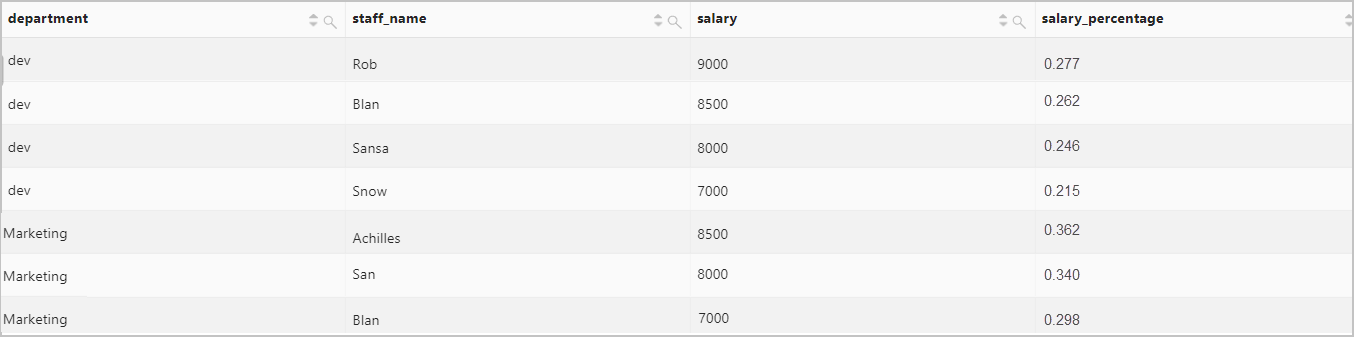## cume_dist function

Calculates the cumulative distribution of each value in a partition. The result is obtained by using division. The numerator is the number of rows whose field values are smaller or equal to the field value of the specified row. The specified row is also counted. The denominator is the total number of rows in the partition. The calculation is based on the order of the rows in the partition. Value range: (0,1].

### Syntax

``````cume_dist() over (
[partition by partition_expression]
[order by order_expression]
)``````

### Parameters

ParameterDescription
partition by partition_expressionSpecifies how the rows are partitioned based on the value of the partition_expression parameter.
order by order_expressionSpecifies how the rows in each partition are ordered. The rows are ordered based on the value of the order_expression parameter.

### Return value type

The double data type.

### Examples

Calculate the cumulative distribution of the size of each object in an OSS bucket named bucket00788.

• Query statement
``````bucket=bucket00788 |
select
object,
object_size,
cume_dist() over (
partition by object
order by
object_size
) as cume_dist
from  oss-log-store``````
• Query result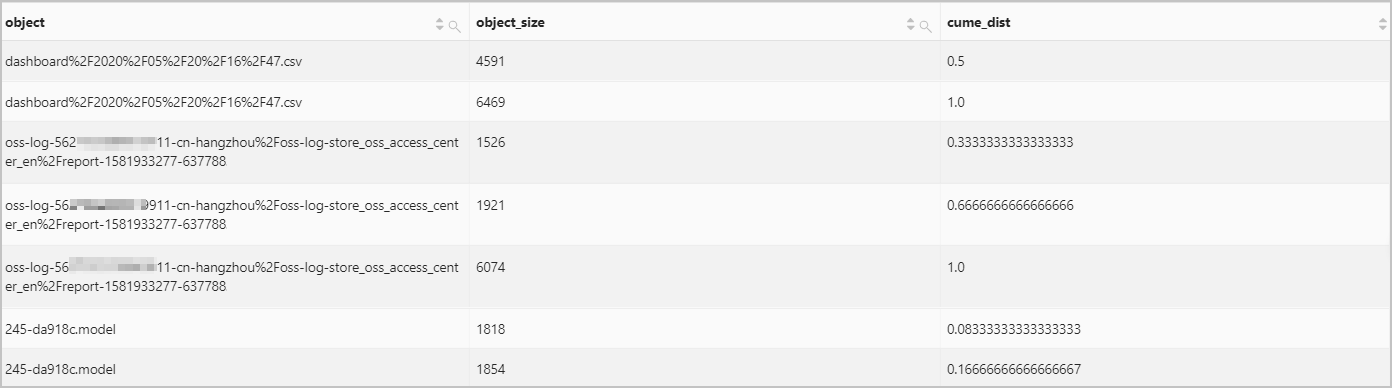## dense_rank function

Calculates the rank of each value in a partition. Rows that have the same field value are assigned the same rank. The ranks are consecutive. For example, if two rows have the same rank of 1, the rank of the next row is 2.

### Syntax

``````dense_rank() over (
[partition by partition_expression]
[order by order_expression]
)``````

### Parameters

ParameterDescription
partition by partition_expressionSpecifies how the rows are partitioned based on the value of the partition_expression parameter.
order by order_expressionSpecifies how the rows in each partition are ordered based on the value of the order_expression parameter.

### Return value type

The bigint data type.

### Examples

Calculate the rank of each employee salary in each department.

• Query statement
``````* |
select
department,
staff_name,
salary,
dense_rank() over(
partition by department
order by
salary desc
) as salary_rank
order by
department,
salary_rank``````
• Query result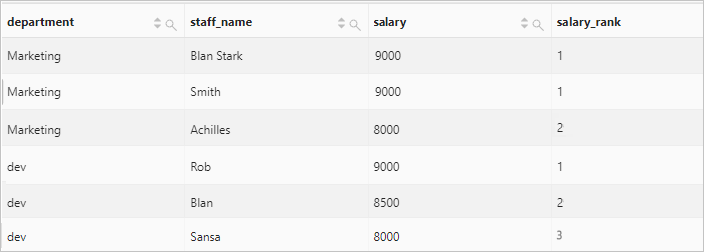## ntile function

Divides the rows in each partition into a number of groups. The number of groups is specified by the N parameter.

### Syntax

``````ntile(n) over (
[partition by partition_expression]
[order by order_expression]
)``````

### Parameters

ParameterDescription
nSpecifies the number of groups.
partition by partition_expressionSpecifies how the rows are partitioned based on the value of the partition_expression parameter.
order by order_expressionSpecifies how the rows in each partition are ordered based on the value of the order_expression parameter.

### Return value type

The bigint data type.

### Examples

Divide the rows in each partition into three groups.

• Query statement
``````object=245-da918c.model |
select
object,
object_size,
ntile(3) over (
partition by object
order by
object_size
) as ntile
from  oss-log-store``````
• Query result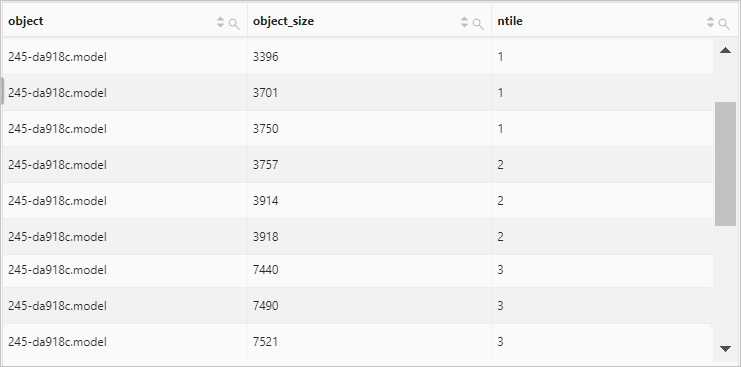## percent_rank function

Calculates the percentage ranking of each row in a partition. The calculation formula is `(rank - 1)/(total_rows - 1)`. In the formula, rank represents the rank of the current row, and total_rows represents the total number of rows in a partition.

### Syntax

``````percent_rank() over (
[partition by partition_expression]
[order by order_expression]
)``````

### Parameters

ParameterDescription
partition by partition_expressionSpecifies how the rows are partitioned based on the value of the partition_expression parameter.
order by order_expressionSpecifies how the rows in each partition are ordered based on the value of the order_expression parameter.

### Return value type

The double data type.

### Examples

Calculate the percentage ranking of the size of each OSS object.

• Query statement
``````object=245-da918c3e2dd9dc9cb4d9283b%2F555e2441b6a4c7f094099a6dba8e7a5f.model|
select
object,
object_size,
percent_rank() over (
partition by object
order by
object_size
) as ntile
FROM  oss-log-store``````
• Query result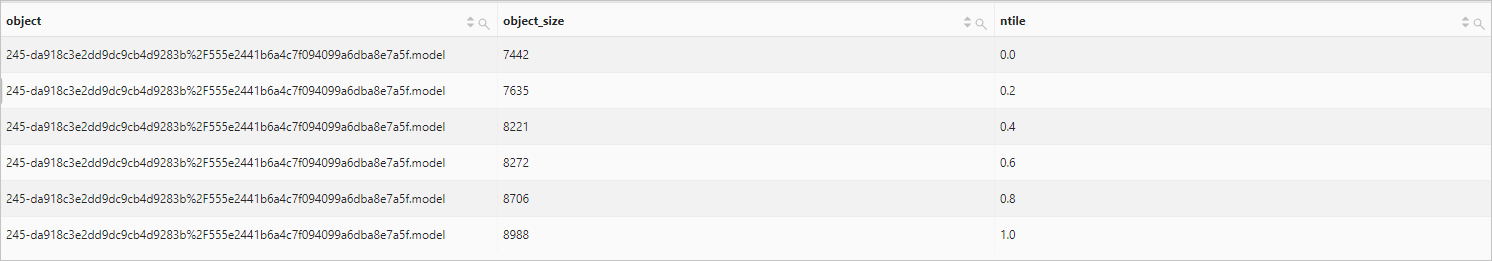## rank function

Calculates the rank of each row in a partition. Rows that have the same field value are assigned the same rank. The ranks are not consecutive. For example, if two rows have the same rank of 1, the rank of the next row is 3.

### Syntax

``````rank() over (
[partition by partition_expression]
[order by order_expression]
)``````

### Parameters

ParameterDescription
partition by partition_expressionSpecifies how the rows are partitioned based on the value of the partition_expression parameter.
order by order_expressionSpecifies how the rows in each partition are ordered based on the value of the order_expression parameter.

### Return value type

The bigint data type.

### Examples

Calculate the rank of each employee salary in each department.

• Query statement
``````* |
select
department,
staff_name,
salary,
rank() over(
partition by department
order by
salary desc
) as salary_rank
order by
department,
salary_rank``````
• Query result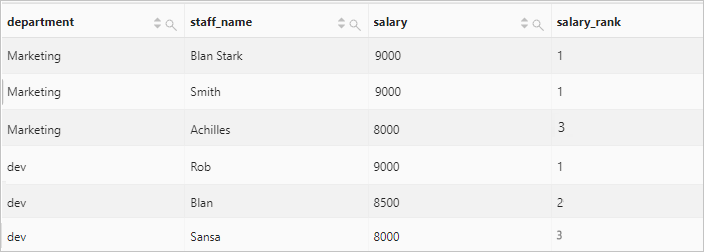## row_number function

Calculate the rank of each row in a partition. The ranks are unique, and start from 1.

### Syntax

``````row_number() over (
[partition by partition_expression]
[order by order_expression]
)``````

### Parameters

ParameterDescription
partition by partition_expressionSpecifies how the rows are partitioned based on the value of the partition_expression parameter.
order by order_expressionSpecifies how the rows in each partition are ordered. The rows are ordered based on the value of the order_expression parameter.

### Return value type

The bigint data type.

### Examples

Calculate the rank of each employee on the salary in each department.

• Query statement
``````* |
select
department,
staff_name,
salary,
row_number() over(
partition by department
order by
salary desc
) as salary_rank
order by
department,
salary_rank``````
• Query result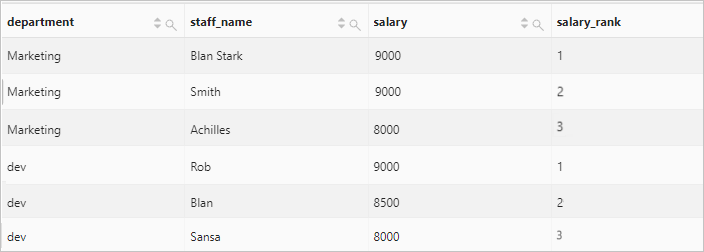## first_value function

Returns the value of the specified field in the first row of each partition.

### Syntax

``````first_value(x) over (
[partition by partition_expression]
[order by order_expression]
[frame]
)``````

### Parameters

ParameterDescription
xThe field name. The field can be of any data type.
partition by partition_expressionSpecifies how the rows are partitioned based on the value of the partition_expression parameter.
order by order_expressionSpecifies how the rows in each partition are ordered based on the value of the order_expression parameter.
frameSpecifies a subset of each partition. Example: `range between unbounded preceding and unbounded following`.

### Return value type

The data type is the same as the data type of the x parameter.

### Examples

Return the minimum size of each object in the specified OSS bucket.

• Query statement
``````bucket :bucket90 |
select
object,
object_size,
first_value(object_size) over (
partition by object
order by
object_size
range between unbounded preceding and unbounded following
) as first_value
from  oss-log-store``````
• Query result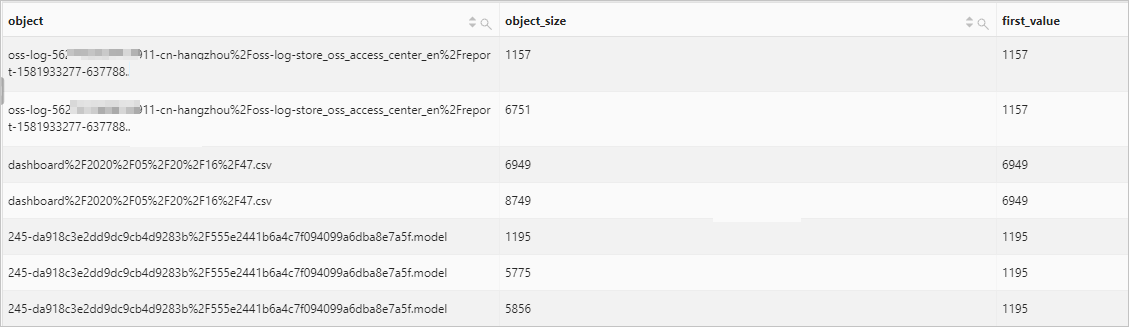## last_value function

Returns the value of the specified field in the last row of each partition.

### Syntax

``````last_value(x) over (
[partition by partition_expression]
[order by order_expression]
[frame]
)``````

### Parameters

ParameterDescription
xThe field name. The field can be of any data type.
partition by partition_expressionSpecifies how the rows are partitioned based on the value of the partition_expression parameter.
order by order_expressionSpecifies how the rows in each partition are ordered based on the value of the order_expression parameter.
frameSpecifies a subset of each partition. Example: `range between unbounded preceding and unbounded following`.

### Return value type

The data type is the same as the data type of the x parameter.

### Examples

Return the maximum size of each object in the specified OSS bucket.

• Query statement
``````bucket :bucket90 |
select
object,
object_size,
last_value(object_size) over (
partition by object
order by
object_size
range between unbounded preceding and unbounded following
) as last_value
from  oss-log-store``````
• Query result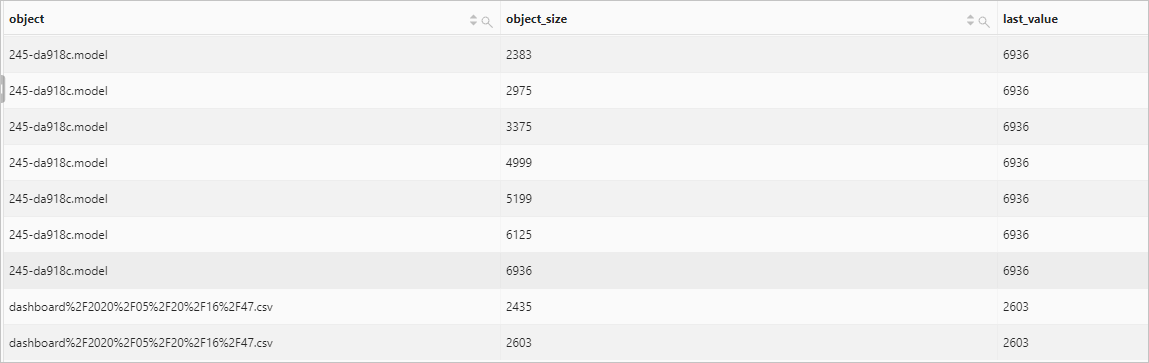## lag function

Returns the value of the specified field in the row that is at the specified offset before the current row in each partition. The offset is specified by the offset parameter.

### Syntax

``````lag(x,offset,defaut_value) over (
[partition by partition_expression]
[order by order_expression]
[frame]
)``````

### Parameters

ParameterDescription
xThe field name. The field can be of any data type.
offsetThe offset before the current row in a partition. If the value of the offset parameter is 0, the value of the specified field in the current row is returned.
defaut_valueIf no row exists at the specified offset before the current row, the value of the defaut_value parameter is returned.
partition by partition_expressionSpecifies how the rows are partitioned based on the value of the partition_expression parameter.
order by order_expressionSpecifies how the rows in each partition are ordered based on the value of the order_expression parameter.
frameSpecifies a subset of each partition. Example: `range between unbounded preceding and unbounded following`.

### Return value type

The data type is the same as the data type of the x parameter.

### Examples

Count the daily unique visitors (UVs) to your website and calculates the percentage of the increase in UVs over the previous day.

• Query statement
``````* |
select
day,
UV,
UV * 1.0 /(lag(UV, 1, 0) over()) as diff_percentage
from  (
select
approx_distinct(client_ip) as UV,
date_trunc('day', __time__) as day
from  log
group by
day
order by
day asc
)``````
• Query result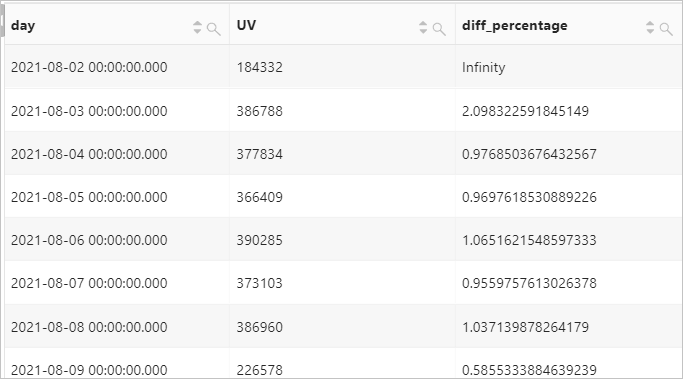Returns the value of the specified field in the row that is at the specified offset after the current row in each partition. The offset is specified by the offset parameter.

### Syntax

``````lead(x,offset,defaut_value) over (
[partition by partition_expression]
[order by order_expression]
[frame]
)``````

### Parameters

ParameterDescription
xThe field name. The field can be of any data type.
offsetThe offset after the current row in a partition. If the value of the offset parameter is 0, the value of the specified field in the current row is returned.
defaut_valueIf no row exists at the specified offset after the current row, the value of the defaut_value parameter is returned.
partition by partition_expressionSpecifies how the rows are partitioned based on the value of the partition_expression parameter.
order by order_expressionSpecifies how the rows in each partition are ordered based on the value of the order_expression parameter.
frameSpecifies a subset of each partition. Example: `range between unbounded preceding and unbounded following`.

### Return value type

The data type is the same as the data type of the x parameter.

### Examples

Count the hourly unique visitors (UVs) to your website on 2021-08-26 and calculates the difference in percentage between UVs of two consecutive hours.

• Query statement
``````* |
select
time,
UV,
UV * 1.0 /(lead(UV, 1, 0) over()) as diff_percentage
from  (
select
approx_distinct(client_ip) as uv,
date_trunc('hour', __time__) as time
from    log
group by
time
order by
time asc
)``````
• Query result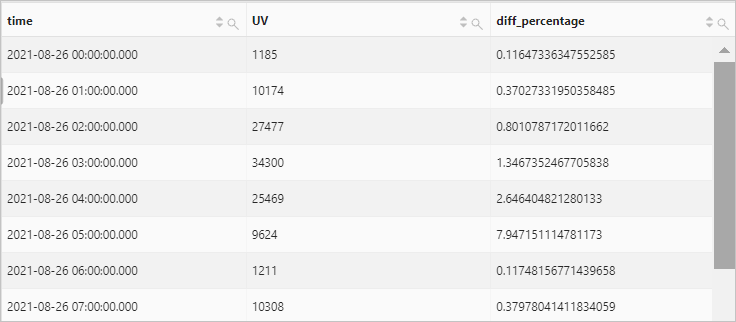## nth_value function

Returns the value of the specified field in the row that is at the specified offset from the beginning of each partition. The offset is specified by the offset parameter.

### Syntax

``````nth_value(x,offset) over (
[partition by partition_expression]
[order by order_expression]
[frame]
)``````

### Parameters

ParameterDescription
xThe field name. The field can be of any data type.
offsetThe offset from the beginning of a partition.
partition by partition_expressionSpecifies how the rows are partitioned based on the value of the partition_expression parameter.
order by order_expressionSpecifies how the rows in each partition are ordered based on the value of the order_expression parameter.
frameSpecifies a subset of each partition. Example: `range between unbounded preceding and unbounded following`.

### Return value type

The data type is the same as the data type of the x parameter.

### Examples

Return the employees whose salary is the second highest in each department.

• Query statement
``````* |
select
department,
staff_name,
salary,
nth_value(staff_name, 2) over(
partition by department
order by
salary desc
range between unbounded preceding and unbounded following
) as second_highest_salary from log``````
• Query result